Electromagnetic Waves Propagation in Graphene Multilayered Structures | OMICS International
Journal of Electrical & Electronic Systems

# Electromagnetic Waves Propagation in Graphene Multilayered Structures

Mohammed M Shabat and Muin F Ubeid*

Department of Physics, Faculty of Science, Islamic University of Gaza, Palestine

*Corresponding Author:
Muin F. Ubeid
Department of Physics, Faculty of Science
Islamic University of Gaza P.O. 108, Gaza
Gaza Strip, Palestinian Authority
E-mail: [email protected]

Received Date: March 08, 2015 Accepted Date: April 25, 2015 Published Date: April 30, 2015

Citation: Ubeid MF, Shabat MM (2015) Electromagnetic Waves Propagation in Graphene Multilayered Structures. J Electr Electron Syst 4:143. doi:10.4172/2332-0796.1000143

Copyright: © 2015 Ubeid MF, et al. This is an open-access article distributed under the terms of the Creative Commons Attribution License, which permits unrestricted use, distribution, and reproduction in any medium, provided the original author and source are credited.

Visit for more related articles at Journal of Electrical & Electronic Systems

#### Abstract

In this work, the reflected, transmitted, and loss powers due to the interaction of electromagnetic waves with graphene-dielectric structures are analyzed theoretically and numerically. The properties of the graphene material are given in detail and the required equations for its main parameters are defined. The formulations for the transverse electric waves case are provided. A recursive method is used to calculate the overall reflection and transmission coefficients of the structure. The reflected, transmitted, and loss powers are determined using these coefficients. In the numerical results, the mentioned powers are computed as a function of wavelength, angle of incidence, and dielectric thickness when graphene thickness changes.

#### Keywords

Graphene; Multilayered structure; Reflection; Transmission; Wavelength

#### Introduction

Graphene film is a monolayer of carbon atoms packed into a dense honeycomb crystal structure that can be viewed as an individual atomic plane extracted from graphite [1,2]. Graphene has high mobility and optical transparency, in addition to flexibility, robustness and environmental stability. It is remarkably strong for its very low weight (100 times stronger than steel), and it conducts heat and electricity. Because it is virtually two-dimensional, it interacts oddly with light and with other materials . While scientists had theorized about graphene for decades, it was first produced in the lab in 2004 . Despite its short history, graphene has already revealed a cornucopia of new physics and potential applications [5-8]. Andre Geim and Konstantin Nevoselov at the university of Manchester won the Nobel prize in physics in 2010 “for groundbreaking experiments regarding the two-dimensional material graphene” .

Researches on graphene-based structures have been developed quickly in both theoretical and experimental applications. Lee et al.  have investigated dynamic behavior of multilayer grapheme via supersonic projectile penetration. Liu et al.  have shown that an enhancement of graphene absorption is observed when the graphene monolayer is placed on the top or within dielectric mirrors. Zhu et al.  have studied optical transmittance of multilayer graphene. Singh et al.  have reviewed optomechanical coupling between a multilayer graphene mechanical resonator and a superconducting microwave cavity. Khan et al.  have demonstrated that, the optimized mixture of graphene and multilayer graphene, produced by the high-yield inexpensive liquid-phase-exfoliation technique, can lead to an extremely strong enhancement of the cross-plane thermal conductivity of the compsite. Iorsh et al.  have proposed a new class of hyperbolic metamaterials for THz frequencies based on multilayer graphene structures. Min et al.  have shown the electronic structure of multilayer graphene. Rast et al.  have numerically analyzed a composite layered structure for, tunable, low-loss plasmon resonators, which consists of a noble metal thin film coated in graphene and supported on a hexagonal boron nitride substrate.

This paper is interested in transmission and reflection of electromagnetic waves by a graphene/dielectric periodic structure consisting of N periods. We consider the structure is embedded in vacuum and a monochromatic s-polarized plane electromagnetic wave is obliquely incident on it. The electric and magnetic fields are determined in each region using Maxwell’s equations. Then Snell’s law is applied and the boundary conditions are imposed at each interface to obtain the reflection and transmission coefficients. The reflected and transmitted powers of the structure are presented in terms of these coefficients. In the numerical analysis a recursive method [18,19] is used to calculate the mentioned powers as a function of wavelength, angle of incidence and the slab thickness when the graphene thickness changes. To check the results of the analysis used in these calculations, the conservation law of energy given in [20,21] is checked and it is clear that it is satisfied for all examples.

#### Theory

The considered waveguide structure consists of a pair of graphene (ε2, μ0) and dielectric (ε3, μ0) materials bounded by two half free spaces (ε0, μ0). The letter ε stands for permittivity of the related materials and the subscripts 2, 3 refer to region 2 and 3 and μ0 is the permeability of free space. A perpendicular polarized plane wave in region 1 is incident on the plane z = 0 at some angle θ relative to the normal to the boundary (Figure 1).

Figure 1: Wave propagation through a structure consisting of a pair of graphene and dielectric embedded between two half free spaces.

The electric field in each region is [22, 23]: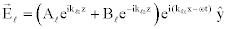(1)

To find the corresponding magnetic field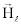, we start with Maxwell’s equation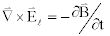, substituting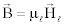and solving foryield: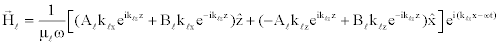(2)

Where Al and Bl are the amplitude of forward and backward traveling waves (l= 1, 2, 3, 4),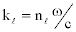is the wave number inside the material and nl is the refractive index of it. Also the subscripts x and z represent the x- and z-components of the related wave number, respectively.

Matching the boundary conditions for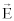and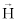fields at each layer interface, that is at z=0,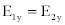and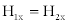and so on.

This yields six equations with six unknown parameters [22,23]:

A1 + B1 = A2 + B2 (3)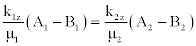(4)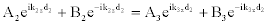(5)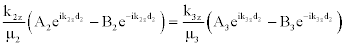(6)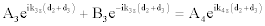(7)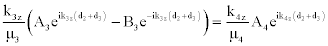(8)

Where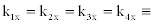Snell’s law and: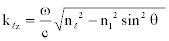(9)

Fresnel coefficients (interface reflection and transmission coefficients r, t respectively) for perpendicular polarized light are given by :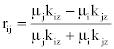(10)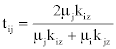(11)

Where i, j correspond to any two adjacent media.

The reflection and transmission coefficients R and T respectively of the structure are given by [25,26]: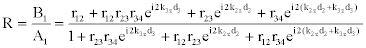(12)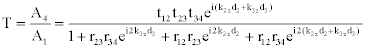(13)

The reflectance R’ and transmittance T’ of the structure are given by: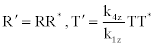(14)

Where R* and T * are the complex conjugate of R and T respectively. The law of conservation of energy is given by [20,21]: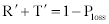(15)

where Ploss is the loss power due to losses in the graphene slab.

For n’-layers structure shown in Figure 2 R and T are calculated as follows [26,27]: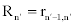(16)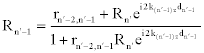(17)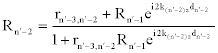(18)

Figure 2: Oblique incidence of electromagnetic waves on graphene/ dielectric periodic structure embedded in vacuum.

`Continue on the same procedure until R2 is reached which is the reflectance of the structure as a whole.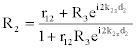(19)

The same procedure is performed for T2 :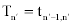(20)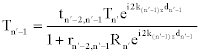(21)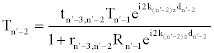(22)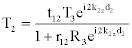(23)

Where d2, dn’–1 and dn’–2 are thicknesses of layers 2, n’-1and n’-2, respectively.

For graphene in regions 2, 4, 6 …, the complex refractive index is given by [28-30]: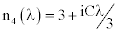(24)

where C is 5.446 μm–1, λ is the incident wavelength and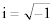Numerical results and applications

In this section, the reflected, transmitted and loss powers of the strucure described in Figure 2 are calculated numerically as a function of wavelength, angle of incidence and dielectric thickness for changing graphene layer thickness. We have used the graphene described in eq. (24) and Fluorite (CaF2)of refractive index 1.434 as a dielectric in each period. Three values the graphene thickness are considered [dg = .34 nm, .68 nm (2 x .34 nm), .68 nm (3 x .34 nm)]. These thicknesses are reported in . The central wavelength is assumed to be λ0 = 600 nm, the thickness of Fluorite is λ0/2 and the number of periods N = 7. Regions 3, 5, 7 … given in Figure 2 are assumed to be loss-less and the permeabilities of them are equal to the permeability of free space, μ0. These materials do not affected by the magnetic field of incident radiations.

Figure 3 shows the reflected (reflectance), transmitted (transmittance) and loss powers as a function of the wavelength at the incidence angle of 30° when the graphene thickness changes. The wavelength is changed between 100 nm and 1700 nm, this range includes ultraviolet, visible and near infrared. We can see that, the powers generally have oscillatory characteristics in the given wavelength range. The degree of ripples decreases with wavelength for all values of the graphene thicknesses. Moreover, the reflected and transmitted powers decrease while the loss power increases with the graphene thickness.

Figure 4 illustrates the variation of the reflected, transmitted, and loss powers with the angle of incidence for 600 nm wavelength under three values of the graphene thickness. The angle of incidence is changed between 0° and 90° to realize all possible angles of incidence. Clearly the reflected power behaves as an oscillatory increasing function while the transmitted and loss powers show an oscillatory decreasing behavior with the angle of incidence. At 90° the reflected (the transmitted and loss) powers is maximum (minimum) at that angle for any value of the graphene thickness. The role of the graphene is clear at angles below 90°. The reflected and transmitted powers decrease while the loss power increases with the graphene thickness for any angle below 90°.

Figure 5 presents the reflected, transmitted and loss powers against the dielectric thickness at the incident angle of 30°. The dielectric thickness is changed from 0 nm to 400 nm. As it is confirmed from the figure, the powers change periodically with thickness for any value of graphene thickness. The reflected and transmitted powers decrease while the loss power increases with graphene thickness.

#### Conclusions

In this paper, the reflection and transmission characteristics of the electromagnetic radiation propagation through a graphene/dielectric (Fluorite-CaF2) periodic structure are studied in detail with the effect of the graphene material. The required equations for the electric and magnetic fields in each region are derived by Maxwell’s equations. Then Snell’s law is applied and the boundary conditions are imposed to calculate the reflection and transmission coefficients of the structure. Recursive method is used to solve the problem of electromagnetic wave propagation through the structure to obtain the reflected, transmitted, and loss powers. Finally, the mentioned powers as a function of wavelength, angle of incidence and the dielectric thickness etc. are studied numerically to observe the effect of the graphene on them. As it can be seen from the theoretical and the numerical results, if the grapheme thickness changes, the characteristic of the powers will be affected by this change. Numerical examples are already presented to illustrate the paper idea and to prove the validity of the obtained results. Moreover the law of conservation of energy is satisfied throughout the performed computations for all examples.

The results obtained could lead to design new devices, apparatus, components at the millimeter wave, optical, and microwave regimes. Furthermore, these results open a way to think how the availability of the graphene will change the functionality of future devices through the graphene/dielectric structure.

#### References

Select your language of interest to view the total content in your interested language

### Article Usage

• Total views: 14143
• [From(publication date):
June-2015 - Feb 24, 2020]
• Breakdown by view type
• HTML page views : 10127Can't read the image? click here to refresh